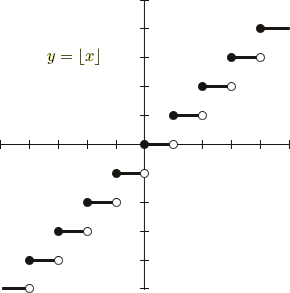# Step Functions

### Greatest Integer Function

The function whose value at any real number x is the greatest integer less than or equal to x is called the greatest integer function (floor function). It is denoted by [x].

f : R → R defined by f(x) = [x]

For example, [2.5] = 2, [3.9] = 3, [−2.1] = −3, [.5] = 0, [−.2] = −1

The domain of the function is R and the range of the function is Z (the set of all integers).### Least Integer Function

The function whose value at any real number x is the smallest integer greater than or equal to x is called the least integer function (ceiling function). It is denoted by ]x[.

f : R → R defined by f(x) = ]x[

For example, ]2.5[ = 3

The domain of the function is R and the range of the function is Z.